# ¶ OPERATORS

## ¶modulus

• Description
• Example
•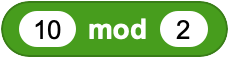The modulus (or "modulo" or "mod") is the remainder after dividing one number by another. e.g.: 10 mod 3 equals 1. Because 10/3 = 3 with a remainder of 1.

The variable num is assigned a random number 1-10. The MOD operation is used to probe the odd/even status of the number. If the remainder of the operation num divided by 2 results in 0, the number is even, else it is odd.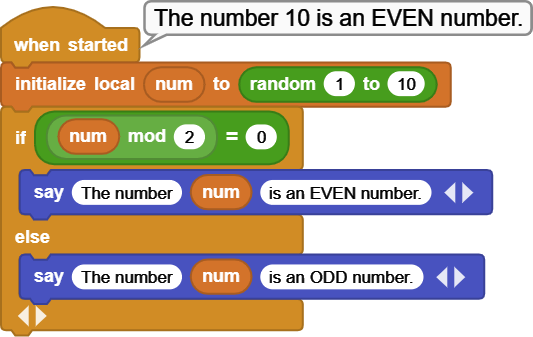## ¶random

• Description
• Example
•Returns a different number between first and second values specified.

One of the common beginners' use of the RANDOM function is to program a HEADS or TAILS implementation.

Here, two variables heads and tails are created, as well as a variable num to store the result of the RANDOM function.

In a loop of 1000 repetitions, heads and tails counts are tallied. Arbitrarily, 1 is counted as heads and 2 is counted as tails. The results are displayed at the end.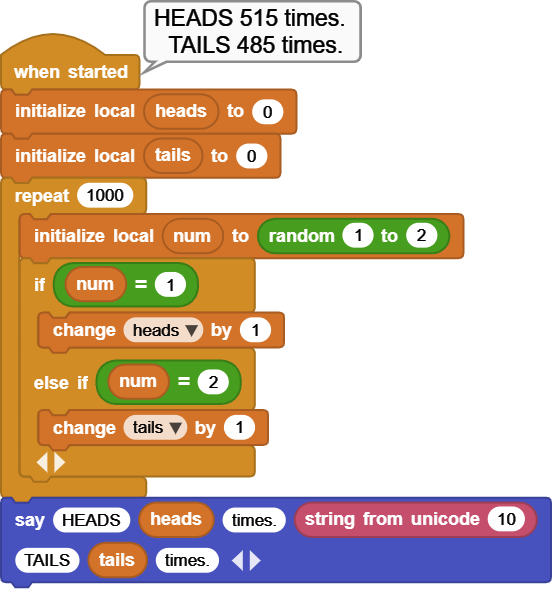## ¶boolean t/f

• Description
• Example
•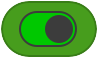Returns true or false depending on the position of the selector, or the binary evaluation of the expression used as input.

You will see this block inside of many other blocks, where it is used to control the flow of the executions and events.

The IF block is a good example to demonstrate the true / false use of this block. The example code is executed two different ways:

First one is a FOREVER loop where the ELSE IF branch of the IF block is set to FALSE - meaning: do NOT evaluate this branch. Therefore, when run, only the first display message is shown. Even though the random block produces 2's, it does not get evaluated, because the else branch is turned off with the FALSE setting.

Also to note is the expression next to the IF. Originally, this position is the true / false block. It gets replaced by the expression num = 1. The binary evaluation of this expression is either true or false depending on what the generated number is. This demonstrates that expressions that evaluate to true or false can be used in place of this block.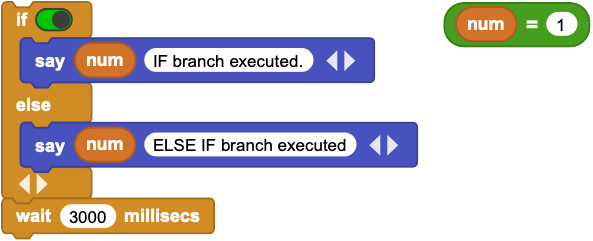In the second one, the FOREVER loop is removed, and the ELSE branch is set to TRUE - meaning: evaluate this branch, if the IF branch is not true. So, if num is not 1 (meaning it is 2), this branch will execute and second message will be shown.

Since the random block generates 1's and 2's, the corresponding message is displayed depending on the number generated.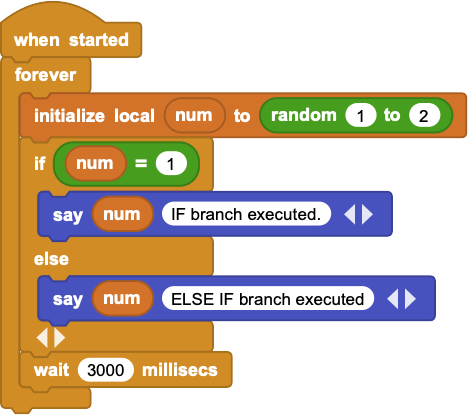## ¶boolean not

• Description
• Example
•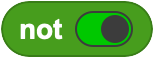Reverses the logical value associated with the expression on which it operates.
As shown, NOT will return FALSE if the slider is set to TRUE, and TRUE if the slider is set to FALSE.

It is also possible to substitute an expression that evaluates to a boolean true or false into the input slot. In that case, the block will evaluate the expression and depending on the result, return the opposite boolean value.

A variable called string is set to "MicroBlocks is great", and a variable called expression is set to "length of string = length of string".
The boolean evaluation of the expression is obviously TRUE.
When the boolean NOT is applied to the expression result, it is changed to the opposite: FALSE. All values are displayed.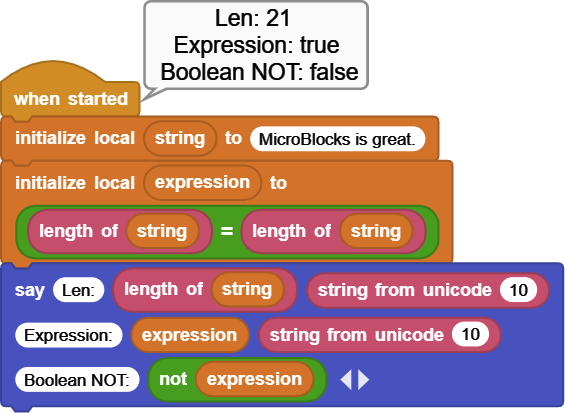## ¶boolean and

• Description
• Example
•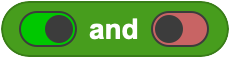Returns true only if either of its inputs are true and returns false otherwise.
It should be noted that since both conditions are required to be true for a true result, if a false is detected in the first condition, there is no need to evaluate the second one; false is returned immediately.

Two variables tuesday and raining are set to true.
Then we check to see if we need an umbrella by evaluating if it is Tuesday and it is raining. Both conditions have to be true for us to need an umbrella.
Play with settings and decidde yourself.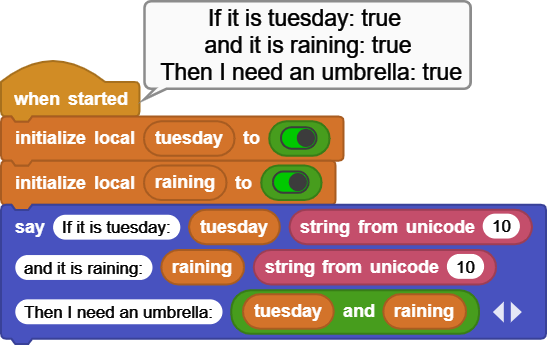## ¶boolean or

• Description
• Example
•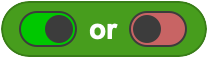Returns true if either or both operands is true and returns false otherwise.

Similar to the boolean_AND but opposite of it, here only one of the two conditions have to be true for a true result. So if the first condition is true, there is no need to check the second one.

In this example we are randomly generating two numbers in the range of 1 - 5. Then we check to see if either one of the two numbers is equal to the number we are looking for.

OR block is used to verify the result of the comparison. All values are reported.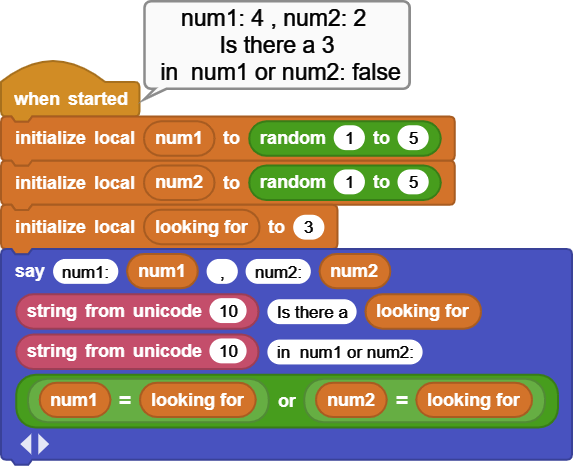## ¶is type

• Description
• Example
•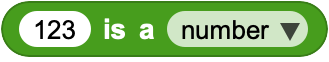Returns true if the input expession on the left side matches the data type menu selection made on the right side.

Data types are important when programming. While an environment like MicroBlocks makes it easy for the beginner users by taking care of any data type conversions, it is still possible to be fooled visually when comparing variables whose values look the same. See example provided.

Two variables data1 and data2 are set to similar looking values:

``````	data1 is set to number 2
data 2 is set to string "2".
``````

IS TYPE block is used to verify the types of the variables. Even though they look the same, they are of DIFFERENT types.

Then we do arithmetic with both fields by adding 1 to each.

Since the data1 is already a number, no problems there.
data2 is a string, but has no visible alphabetic characters in front of the "2".
Therefore, MicroBlocks is able to convert it to number 2 for us, and do the addition.

At the end, when we compare the values of data1+1 to data2+1, we get a TRUE because both of expressions evauate to number 3.

Now try the same after placing any letter into the first input field of the join block for data2, and see what results you will get.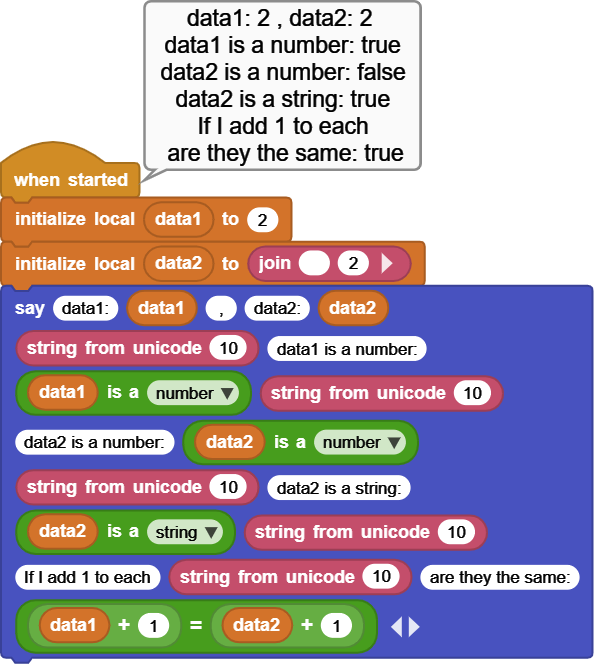This section contains some blocks that represent bitwise operations.

The bitwise operators are similar to the logical operators (booleans), except that they work on binary representations of data.
Bitwise operators are used to change individual bits in an operand.
Both operands associated with the bitwise operator must be integers.

To make it easier to follow the details and examples presented below, a reference table detailing Byte Positional values in decimal and hex, have been provided.

Byte Positions and Values

Byte Position: 7 6 5 4 3 2 1 0
Decimal Value: 128 64 32 16 8 4 2 1
Hex 80 40 20 10 08 04 02 01

### ¶ Anatomy of a binary number:

A binary number is a number expressed in the base-2 numeral system or binary numeral system, which uses only two symbols: typically "0" (zero) and "1" (one).

A byte is an eight bit binary number. Each bit is either a 0 or a 1.

To determine the decimal value of a binary number of eight bits, refer to the Byte Position table and add all decimal values for columns where the binary number has a 1.

Example:

Binary Number: 0010 0110     Decimal Value: 38

Explanation:

Byte Position: 7 6 5 4 3 2 1 0
Decimal Value: 128 64 32 16 8 4 2 1
Binary Number 0 0 1 0 0 1 1 0

Total all the decimal values with a binary 1 in that column:
Decimal Values: 32 + 4 + 2 = 38

## ¶hex

• Description
• Example
•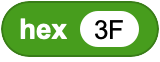Converts a hexadecimal string to a decimal number.

As a quick intro, hex numbers consist of 0 - 9 and A, B, C, D, E, F.
Thus, hex(3F) evalutes to 63 in decimal.

Note that HEX block inputs have to be of type STRING, since part of the hex numbers consist of letters A -F.

hex to decimal conversion is a very common operation. Each hexadecimal digit represents exactly four bits, so one byte is represented by two digits. That simple mapping between digits and bytes makes hexadecimal useful when working with certain kinds of data. For example, RGB color codes.

Dec - Hex - Bin Number Conversion

Decimal Hex Binary Decimal Hex Binary
0 00 0000 8 08 1000
1 01 0001 9 09 1001
2 02 0010 10 0A 1010
3 03 0011 11 0B 1011
4 04 0100 12 0C 1100
5 05 0101 13 0D 1101
6 06 0110 14 0E 1110
7 07 0111 15 0F 1111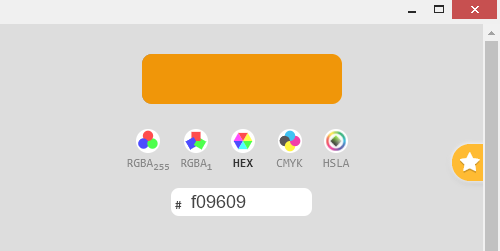Chrome browser's "Color For Developer's" extension enables one to pick a color from a suite of color palettes used on the web. The color is displayed in RGB format as three hex numbers.

If we want to set the CLUE rgbLED color using the Neopixel Library blocks, we need to convert the RGB values into decimal equivalents.

Example program accepts three hex numbers and displays their decimal equivalents. It then sets the NeoPixel RGB values accordingly.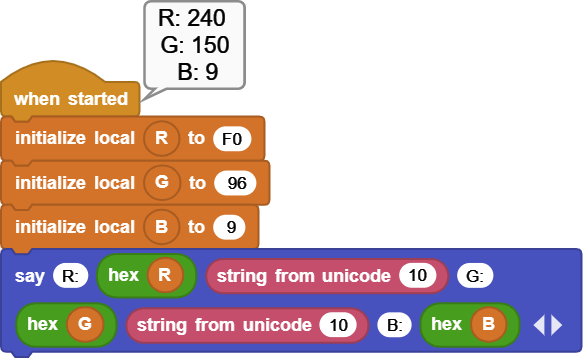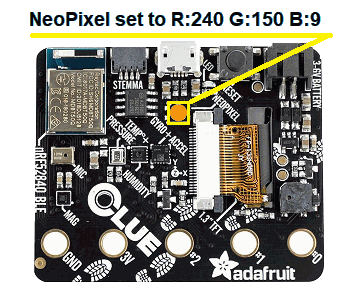## ¶bitwise and

• Description
• Example
•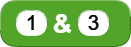The AND operator compares two bits and generates a result of 1 if both bits are 1; otherwise, it returns 0.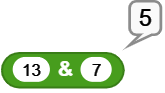Explanation:

Byte Position: 7 6 5 4 3 2 1 0
Decimal Value: 128 64 32 16 8 4 2 1
13 0 0 0 0 1 1 0 1
7 0 0 0 0 0 1 1 1
Result = 5 0 0 0 0 0 1 0 1
• Bitwise AND compares all the bit positions of both numbers and reports the ones where both are set to 1.
• Between #13 and #7, the positions where both numbers have a 1 are positions 2 and 0.
• The decimal value of position 2 is 4, and of position 0 is 1.
• Therefore the operation reports 4 + 1 = 5.

## ¶bitwise or

• Description
• Example
•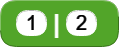The OR operator compares two bits and returns 1 if either or both of the bits are 1 and it gives 0 if both bits are 0.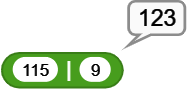Explanation:

Byte Position: 7 6 5 4 3 2 1 0
Decimal Value: 128 64 32 16 8 4 2 1
115 0 1 1 1 0 0 1 1
9 0 0 0 0 1 0 0 1
Result = 123 0 1 1 1 1 0 1 1
• Bitwise OR compares all the bit positions of both numbers and reports the ones where either are set to 1.
• Between #115 and #9, the position where either numbers have a 1 are positions 6,5,4,3,1, and 0.
• The decimal value of position 2 is 4, and of position 0 is 1.
• Therefore the operation reports 64 + 32 + 16 + 8 + 2 + 1 = 123.

## ¶bitwise xor

• Description
• Example
•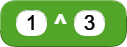The EXCLUSIVE-OR operator compares two bits and generates a result of 1 if the bits are complementary (bit is set in one operand but not both); otherwise, it returns 0.

Another way to think of the XOR operation is that it will reverse the bit positions where the second operand has a 1. All others will be copied as the same.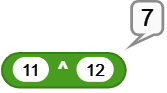Explanation:

Byte Position: 7 6 5 4 3 2 1 0
Decimal Value: 128 64 32 16 8 4 2 1
11 0 0 0 0 1 0 1 1
12 0 0 0 0 1 1 0 0
Result = 7 0 0 0 0 0 1 1 1
• Bitwise XOR compares all the bit positions of the first operand and reports the opposite of where the second operand has a 1.
• #12 has a 1 in positions 3 and 2. Bits in the same positions of #11 ( 10 ) will be reversed, all other bits of #11 will be copied as they are. For position 3 and 2 reversed, we get 01.
• We end up with 0000 0111 for the result. Positions 2, 1, and 0 are 1.
• The decimal value of position 2 is 4, position 1 is 2, and position 0 is 1.
• Therefore the operation reports 4 + 2 + 1 = 7.

## ¶bitwise not

• Description
• Example
•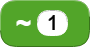The NOT (or COMPLEMENT) operator is used to invert (BIT FLIP) all of the bits of the operand.
When the highest position bit of a number becomes 1, that number is considered a negative number.

Actual computer representation of an signed integer in MicroBlocks is 31 bits long. The high order bit #31 is a sign bit. This allows numbers in the range of -1073741824 to 1073741823.

When the sign bit is 0 the number is positive; and when it is 1, the number is negative.

NOT operation reverses all bits and turns positive numbers into negative ones and vice versa.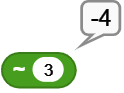Explanation:

NOT operation reverses all bits and turns positive numbers into negative ones.

Decimal 3 ( Binary: 0011 ) becomes decimal -4 ( Binary: 1100 ).

NOTE: In the 4-bit example used here, the high order bit (bit-3) is the sign bit.

## ¶bitwise left shift

• Description
• Example
•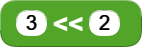The left operands value is moved left by the number of bits specified by the right operand.

The bits are moved to the left, the far left bit is discarded, and the rightmost bit is assigned a value of 0.
For every bit position moved to the left, this operation is the binary equivalent of multiplying by 2.

REMEMBER
For signed positive numbers that naturally have a 0 bit in the most significant position of their binary representation, this operation will eventually turn that into a 1 bit, thus changing the number to a negative one.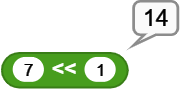Decimal number 7 is Left shifted 1 bit position.
Bit positions of the result are: positions 3, 2, and 1; with corresponding values of 8, 4, 2.
Therefore the operation reports: 8 + 4 + 2 = 14.

Explanation:

Byte Position: 7 6 5 4 3 2 1 0
Decimal Value: 128 64 32 16 8 4 2 1
7 0 0 0 0 0 1 1 1
14 0 0 0 0 1 1 1 0

## ¶bitwise right shift

• Description
• Example
•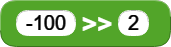The left operands value is moved right by the number of bits specified by the right operand.

The bits are moved to the right, the far right bit is discarded, and the leftmost bit is assigned a copy of the sign bit. This is called an arithmetic shift.
For every bit position moved to the right, this operation is the binary equivalent of dividing by 2.

REMEMBER
For signed negative numbers that naturally have a 1 bit in the most significant position of their binary representation, this operation will maintain the sign bit and successively produce negative numbers.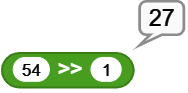Decimal number 7 is Left shifted 1 bit position.
Bit positions of the result are: positions 4, 3, 1, and 0; with corresponding values of 16, 8, 2, and 1.
Therefore the operation reports: 16 + 8 + 2 + 1 = 27.

Explanation:

Byte Position: 7 6 5 4 3 2 1 0
Decimal Value: 128 64 32 16 8 4 2 1
54 0 0 1 1 0 1 1 0
27 0 0 0 1 1 0 1 1